# GSEB Class 9 Maths Notes Chapter 15 Probability

This GSEB Class 9 Maths Notes Chapter 15 Probability covers all the important topics and concepts as mentioned in the chapter.

## Probability Class 9 GSEB Notes

In everyday life, we come across words like ‘probably’, ‘doubt’, ‘most probably’, ‘chances’, etc. These word involve an element of uncertainty.

Uncertainty and Chance:
In our day-to-day life, there are certain events for which we can predict with certainty such as the sun rising in east or an object thrown upwards coming down to earth, etc. But, there are certain events for which we know all possible outcomes of the event when a trial is carried out, but we cannot predict with certainty that which outcome will occur and which will not occur. This uncertainty gives rise to chance and its mathematical study leads to Probability.

The scientific approach to probability was initiated by French mathematicians Pascal and Fermat in the seventeenth century. The study of probability began initially with the chance – dependent games of gambling. Later on it developed to its fully mathematical form and is now applied in large number of fields.

Trial:
Trial is an action which results in one or several outcomes.

Event:
An event for an experiment is the collection of some outcomes of the experiment. Events are usually denoted by capital letters A, B, C,… etc.

Mutually exclusive events:
For events A and B, if there is no common trial between them, then A and B are called mutually exclusive events.

Exhaustive events:
A group of events is called exhaustive events if all the events together consume all the possible trials.

Empirical probability:
Empirical probability is based on the outcomes of an experiment as we observe them. The probability calculated on the observations of an experiment is called experimental or empirical probability.
In this chapter, we shall be finding the empirical probability, though we will use the word ‘probability’ for convenience.Probability:
Probability of any event is given by the ratio of the number of trials in which that event occurs to the total number of triads of the experiment. Probability of occurance of an event A is denoted by P (A).

P (A) = $$\frac{\text { Number of trials in which event A occured }}{\text { The total number of trials }}$$

Properties of probability:

• The probability of any event lies between 0 and 1 including both. So, 0 ≤ P(A) ≤ 1, for any event A.
• The sum of the probabilities of all mutually exclusive and exhaustive events is 1.
• The probability of an impossible event is 0 and the probability of a certain event is 1.

Example 1:
A coin is tossed 1000 times with the following frequencies:
Compute the probability for each event.
Since the coin Is tossed 1000 times, the total number of trials Is 1000. Let us call the events of getting a head and of getting a tall as E and F, respectively. Then, the number of times E occures, i.e., the number of times a head comes up is 455.

So, the probability of E = $$\frac{\text { Number of heads }}{\text { Total number of trials }}$$
∴ P (E) = $$\frac{455}{1000}$$ = 0455

Similarly, the probability of the event of getting

a trials = $$\frac{\text { Number of tails }}{\text { Total number of trials }}$$
∴P(F) = $$\frac{545}{1000}$$ = 0.545
Note that In the example above.
P(E) + P(F) = 0.455 + 0.545 = 1, and E and F are the only two possible outcomes of each trial.

Example 2:
Two coins are tossed simultaneously 500 times, and we get
Find the probability of occurrence of each of these events.
Let us denote the events of getting two heads, one head and no head by E1, E2 and E3, respectively. Then,
P(E1) = $$\frac{105}{500}$$ = 0.21
P(E2) = $$\frac{275}{500}$$ = 0.55
P(E3) = $$\frac{120}{500}$$ = 0.24
Observe that P (E1) + P (E2) + P (E3) = 1. Also
E1, E2 and E3 cover all the outcomes of a trial.

Example 3:
A die is thrown 1000 times with the frequencies for the outcomes 1, 2. 3, 4, 5 and 6 as given in the following table: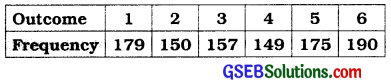Find the probability of getting each outcome.
Let Ei denote the event of getting the outcome i, where i = 1, 2, 3, 4, 5, 6.
Then, Probability of the outcome I
= P(E1)
= $$\frac{\text { Frequency of 1}{\text { Total number of times the die is thrown }}$$
= $$\frac{179}{1000}$$
= 0.179
Similarly, P (E2) = $$\frac{150}{1000}$$ = 0.15
P(E3) = $$\frac{157}{1000}$$ = 0.157
P(E4) = $$\frac{149}{1000}$$ = 0.149
P(E5) = $$\frac{175}{1000}$$ = 0.175
P(E6) = $$\frac{190}{1000}$$ = 0.19

Note that:
P(E1) + P(E2) + P(E3) + P(E4) + P(E5) + P(E6) = 1

Example 4.
On one page of a telephone directory, there were 200 telephone numbers. The frequency distribution of their unit place digit (for example, in the number 25828573, the unit place digit is 3) is given in the following table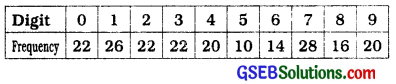Without looking at the page, the pencil is placed on one of these numbers, i.e., the number is chosen at random. What is the probability that the digit in its unit place is 6?
The probabifity of digIt 6 being In the unit place
= $$\frac{\text { Frequency of 1}{\text { Total number of Selected telephone number }}$$
= $$\frac{14}{200}$$ = 0.07
You can similarly obtain the empirical probabilities of the occurrence of the numbers having the other digits In the unit place.

Example 5:
The record of a weather station shows that out of the past 250 consecutive days, its weather forecasts were correct 175 times.
(i) What is the probability that on a given day it was correct?
(ii) What is the probability that it was not correct on a given day?
The total number of days for which the record is available = 250
(i) P(the forecast was correct on a given day)
Number of days when the forecast was correct
= $$\frac{\text { Number of days when the forecast was correct}{\text { Total number of days for which the record Is available }}$$
= $$\frac{14}{250}$$ = 0.7

(ii) The number of days when the forecast was not correct = 250 – 175 = 75
So, P (the forecast was not correct on a given day) = $$\frac{75}{250}$$ = 0.3

Notice that:
P (forecast was correct on a given day) + P (forecast was not correct on a given day)
= 0.7 + 0.3 = 1Example 6:
A tyre manufacturing company kept a record of the distance covered before a tyre needed to be replaced. The table shows the results of 1000 cases: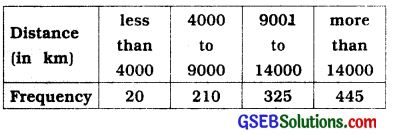If you buy a tyre from this company, what is the probability that:
(i) it will need to be replaced before it has covered 4000 km?
(ii) it will last more than 9000 km?
(iii) it will need to be replaced after it has covered somewhere between 4000 km and 14000 km?
(i) The total number of trials = 1000.
The frequency of a tyre that needs to be replaced before it covers 4000 km is 20.
So, P (tyre to be replaced before it covers 4000 km) = $$\frac{20}{1000}$$ = 0.02

(ii) The frequency of a tyre that will last more than 9000 km is 325 + 445 = 770
So, P(tyre will last more than 9000 km) = $$\frac{770}{1000}$$

(iii) The frequency of a tyre that requires replacement between 4000 km and 14000 km is 210 + 325 = 535.
So, P (tyre requires replacement between 4000km and 14000 km) = $$\frac{535}{1000}$$ = 0.535

Example 7:
The percentage of marks obtained by a student in the monthly unit tests are given below: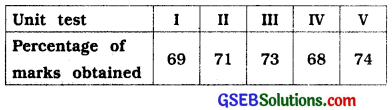Based on this data, find the probability that the student gets more than 70 % marks in a unit test.
The total number of unit tests held is 5.
The number of unit tests In which the student obtained more than 70 % marks Is 3.
So, P (scoring more than 70 % marks) = $$\frac{3}{5}$$ = 0.6

Example 8:
An insurance company selected 2000 drivers at random (i.e., without any preference of one driver over another) in a particular city to find a relationship between age and accidents. The data obtained are given in the following table: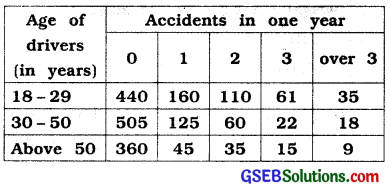Find the probabilities of the following events for a driver chosen at random from the city:
(i) being 18 – 29 years of age and having exactly 3 accidents in one year.
Total number of drivers = 2000.
(i) The number of drivers who are 18 – 29 years old and have exactly 3 accidents In one year is 61.
So, P (driver is 18-29 years old with exactly 3 accidents) = $$\frac{61}{2000}$$
= 0.0305 ≈ 0.031

(ii) being 30 – 50 years of age and having one or more accidents in a year.
The number of drivers 30-50 years of age and having one or more accidents in one year = 125 + 60 + 22 + 18 = 225
So, P (driver is 30-50 years of age and having one or more accidents) = $$\frac{225}{2000}$$
= 0.1125 ≈ 0.113

(iii) having no accidents in one year.
The number of drivers having no accidents in one year = 440 + 505 + 360 = 1305
Therefore, P (drIver with no accident)
= $$\frac{1305}{2000}$$ = 0.653

Example 9:
Consider the frequency distribution table (Table 3, sum no. 4 of “Sums to Enrich ‘Remember’” Chapter 14), which gives the weights of 38 students of a class.
(i) Find the probability that the weight / of a student in the class lies in the interval 46 – 50 kg.
(ii) Give two events in this context, one having probability 0 and the other having probability 1.
The table which is referred in this example is as below:

 Weight (in kg) No. of students 31-35 9 36-40 5 41-45 14 46-50 3 51-55 1 56-60 2 61-65 2 66-70 1 71-75 1 Total 38

(i) The total number of students is 38, and the number of students with weight in the Interval 46-50 kg is 3. So, P (weIght of a student Is In the Interval 46-50 kg) = $$\frac{3}{38}$$ = 0.079

(ii) For Instance, consider the event that a student weighs 30 kg. Since no student has this weight, the probability of occurrence of this event Is O. Similarly, the probability of a student weighing more than 30 kg is $$\frac{38}{38}$$ = 1Example 10:
Fifty seeds were selected at random from each of 5 bags of seeds, and were kept under standardised conditions favourable to germination. After 20 days, the number of seeds which had germinated in each collection were counted and recorded as follows :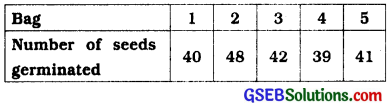What is the probability of germination of
(i) more than 40 seeds in a bag?
(ii) 49 seeds in a bag?
(iii) more that 35 seeds in a bag?
(i) Number of bags in which more than 40 seeds germinated out of 50 seeds is 3. P (germination of more than 40 seeds in a bag) = $$\frac{3}{5}$$ = 0.6
P (germination of 49 seeds in a bag) = $$\frac{0}{5}$$ = 0
So, the required probability = $$\frac{5}{5}$$ = 1.